<h1>定义</h1>

<h2>链剖分</h2>

• 查询，修改链信息
• 随意换根
• 动态连边，删边
• 合并树，分离树
• 动态维护连通性
1. 每一个 Splay 维护的是一条从上到下按在原树中深度严格递增的路径，且中序遍历 Splay 得到的每个点的深度序列严格递增
2. 每个节点包含且仅包含在一个 Splay 中
3. 实边是包含在 Splay 中的，而虚边是节点连向另一个 Splay 的边。

<h1>操作</h1>

<h2>Access 操作</h2>

这是 LCT 的核心操作，其他的操作都是基于此完成的。
这个操作就是在满足 LCT 性质的前提下，将任意一个节点和根节点之间的路径变成一条实链。
下面的图参考YangZhe的论文
一开始我们有一个这样的树（虚线表示虚边）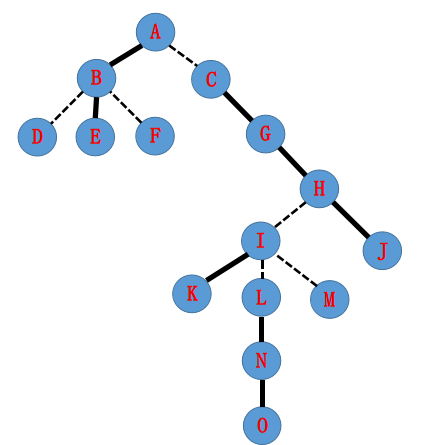那么 LCT 有可能如下图所示（每个框里的元素都在一个 Splay 中）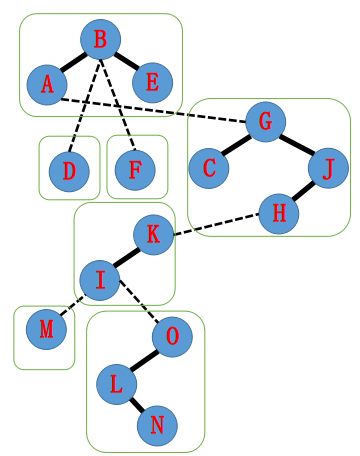现在我们要执行 access(N) 操作了。
由性质 2 可得为了使得 A 到 N 上全是实边，需要使得其他的边变成虚边。
所以可能划分成这样：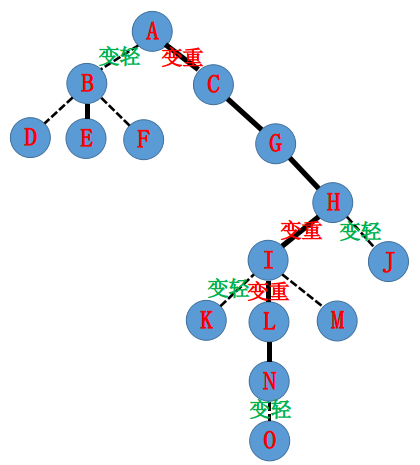怎么实现这一个过程呢？我们可以让 N 点一直跳 Splay 来实现。
首先执行 Splay(N) 将 N 伸展为根节点，为了满足性质二，$N-O$ 要变为轻边。
为了满足性质一：单方面将 N 的右儿子设置为空。
然后变成下图：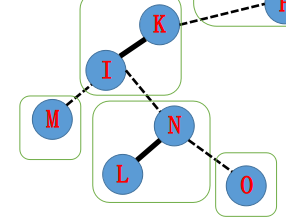我们接着把 N 指向的点 I 也伸展到它所属的 Splay 的根节点，为了维护性质，I 到 K 的边要单方面废除，这时候 I 到 L 可以变成实边了，按照性质 1 将 N 放在 I 的右儿子上。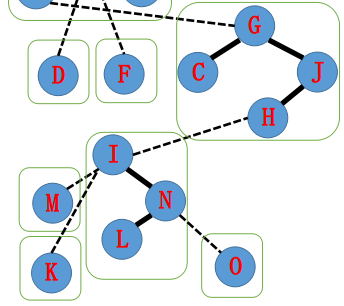多次重复该过程直到路径上全为实边。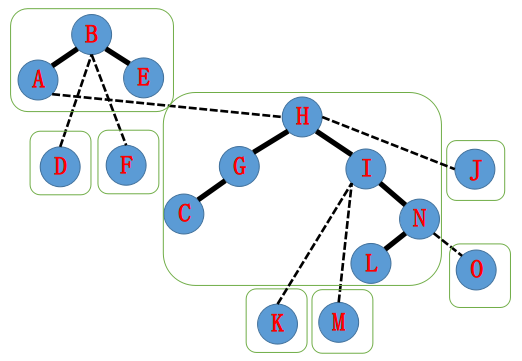归纳一下上述过程：

1. 伸展到当前 Splay 的根节点
2. 交换儿子
3. 更新信息(pushup)
4. 操作点换为轻边指向的父亲，转1
inline void access(int x){
for(int y=0;x;x = f[y = x]){
splay(x);rc = y;pushup(x);
}
}


<h2>makeroot 操作</h2>

钦定新根操作。我们在运用中有可能查询两点路径无论如何不可能在一个 Splay 中，这个时候我们考虑改变树的根而保持树维护信息不变，将其放在一个 Splay 里求解。
首先一定要让 $x$ 和 $root$ 在一个 Splay 里，然后将 $x$ 伸展到根。然后注意到一开始 $x$ 的深度是最大的，那么我们如果翻转整个 Splay，$x$ 就变成了深度最小的节点（即根），所以我们打个标记翻转即可。

inline void reverse(int x){
std::swap(lc,rc);tag[x] ^= 1;
}
inline void makeroot(int x){
access(x);splay(x);reverse(x);
}


<h2>findroot 操作</h2>

查询根节点操作，主要用来判断连通性。
大概就是先让 $x$ 成为 Splay 中的根，然后按照定义一一直走左儿子，最后的那个点一定是深度最小的（即根）。

inline int findroot(int x){
access(x);splay(x);
while(lc) pushdown(x),x=lc;
// splay(v);  某势能分析指出这一句话是要加来保证复杂度的，但是不加貌似没错.....
return x;
}


<h2>split 操作</h2>

我们想将 $x$ 到 $y$ 的路径放入一个 Splay 里，需要借助 makeroot 操作进行实现。
直接将 $x$ 钦定为根，然后将 $y$ 放入和 $x$ 一样的 Splay 里并伸展为 Splay 的根节点，这样我们查询路径信息其实就是查询 $y$ 的信息了 qwq。

inline void split(int x,int y){
makeroot(x);access(y);splay(y);
}


连边操作。特判如果这条边不存在直接加一条虚边。

inline void link(int x,int y){
makeroot(x);
if(findroot(y) != x) f[x] = y;
}


<h2>cut 操作</h2>

删除一条边。注意到这里特判比较多，即特判以下情况：

1. 不存在这条边
2. $x$ 的父亲不是 $y$，即 $x$ 和 $y$ 虽然在一个 Splay 中但是没有连边。
3. $x$ 的右子树非空，这时以 $y$ 为根开始的中序遍历中 $x$ 和 $y$ 不在一起，即没有边。
inline void cut(int x,int y){
makeroot(x);
if(findroot(y) == x && f[x] == y && !rc){
f[x] = childy = 0;
pushup(y);
}
}


此外还要注意 LCT 中的 Splay 和日常写的有些不一样，有些地方是要判断的。
注意 findroot 判断连通性很慢，如果题目只有合并操作的话，建议使用并查集。

<h1>完整代码</h1>

题目链接

#include <algorithm>
#include <iostream>
#include <cstring>
#include <climits>
#include <cstdio>
#include <vector>
#include <cstdlib>
#include <ctime>
#include <cmath>
#include <queue>
#include <stack>
#include <map>
#include <set>

#define Re register
#define LL long long
#define U unsigned
#define lc childx
#define rc childx
#define FOR(i,a,b) for(Re int i = a;i <= b;++i)
#define ROF(i,a,b) for(Re int i = a;i >= b;--i)
#define SFOR(i,a,b,c) for(Re int i = a;i <= b;i+=c)
#define SROF(i,a,b,c) for(Re int i = a;i >= b;i-=c)
#define CLR(i,a) memset(i,a,sizeof(i))
#define BR printf("--------------------n")
#define DEBUG(x) std::cerr << #x << '=' << x << std::endl

const int MAXN = 300000+5;
int f[MAXN],childMAXN,val[MAXN],s[MAXN],tag[MAXN];
bool r[MAXN];

inline bool nroot(int x){
return child[f[x]] == x || child[f[x]] == x;
}

inline void pushup(int x){
s[x] = s[lc]^s[rc]^val[x];
}

inline void reverse(int x){
int t = lc;lc = rc;rc = t;tag[x] ^= 1;
}

inline void pushdown(int x){
if(tag[x]){
if(lc) reverse(lc);
if(rc) reverse(rc);
tag[x] = 0;
}
}

inline void rotate(int x){
int y = f[x],z = f[y];int k = childy==x,w = childx;
if(nroot(y)) childz==y] = x;
childx = y;childy = w;
if(w) f[w] = y;
f[y] = x,f[x] = z;
pushup(y);
}
int st[MAXN];
inline void splay(int x){
int y = x,z;st[z = 1] = y;
// std::stack<int> S;
// S.push(y);
while(nroot(y)) st[++z] = y = f[y];//S.push(y=f[y]);
while(z) pushdown(st[z--]);
//while(!S.empty()) pushdown(S.top()),S.pop();
while(nroot(x)){
y = f[x];z = f[y];
if(nroot(y)) rotate((childy == x) ^ (childz == y) ? x : y);
rotate(x);
}
pushup(x);
}

inline void access(int x){
for(int y = 0;x;x = f[y = x]){
splay(x);rc = y;pushup(x);
}
}

inline void makeroot(int x){
access(x);splay(x);reverse(x);
}

inline int findroot(int x){
access(x);splay(x);
while(lc) pushdown(x),x = lc;
// splay(v);  某势能分析指出这一句话是要加来保证复杂度的，但是不加貌似没错.....
return x;
}

inline void split(int x,int y){
makeroot(x);access(y);splay(y);
}

makeroot(x);
if(findroot(y) != x) f[x] = y;
}

inline void cut(int x,int y){
makeroot(x);
if(findroot(y) == x && f[x] == y && !rc){
f[x] = childy = 0;
pushup(y);
}
}

int N,M;

int main(){
scanf("%d%d",&N,&M);
FOR(i,1,N) scanf("%d",val+i);
FOR(i,1,M){
int opt,x,y;scanf("%d%d%d",&opt,&x,&y);
if(opt == 0) split(x,y),printf("%dn",s[y]);